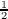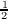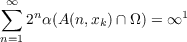#### Vol. 29, No. 3, 1969

 Download this articleFor screen For printingRecent Issues Vol. 325: 1  2 Vol. 324: 1  2 Vol. 323: 1  2 Vol. 322: 1  2 Vol. 321: 1  2 Vol. 320: 1  2 Vol. 319: 1  2 Vol. 318: 1  2Online Archive Volume: Issue:The Journal Subscriptions Editorial Board Officers Contacts Submission Guidelines Submission Form Policies for Authors ISSN: 1945-5844 (e-only) ISSN: 0030-8730 (print) Special Issues Author Index To Appear Other MSP Journals
Rational approximation on certain plane sets

### Thomas Anthony Mc Cullough

Vol. 29 (1969), No. 3, 631–640
##### Abstract

Let K be a compact subset of the complex plane and let Ω denote its complement. In 1966 Vituskin  proved the following generalization of Mergelyan’s celebrated theorem on rational approximation .

Theorem. (Vituskin). If each boundary point of K is a boundary point of some component of Ω then A(K), the subset of continuous functions on K which are analytic on the interior of K, is the same as R(K), the uniform closure of the rational functions with poles in Ω.

The complexity of Vituskin’s techniques justifies the development of alternate approaches to this problem. For a complete discussion of Vituskin’s techniques and results see . The alternate approach we have in mind exploits a recent result of Garnett and Glicksberg . Namely, R(K) = A(K) if they have the same representing measures for each point φ K.

We are unable, at present, to prove Vituskin’s result. However, if Ωi denotes the i-th component of Ω, if A(n,z) denotes the annulus {()n+1 |ξ z|()n}, and if α denotes analytic capacity, then we prove the following

Theorem. If K is such that (1) (K), the boundary of K, has finitely many components and (2) ∂K = { Ωi}∪{x1,x2,}, wherefor each xk, then R(K) = A(K).

Primary: 30.70
##### Milestones